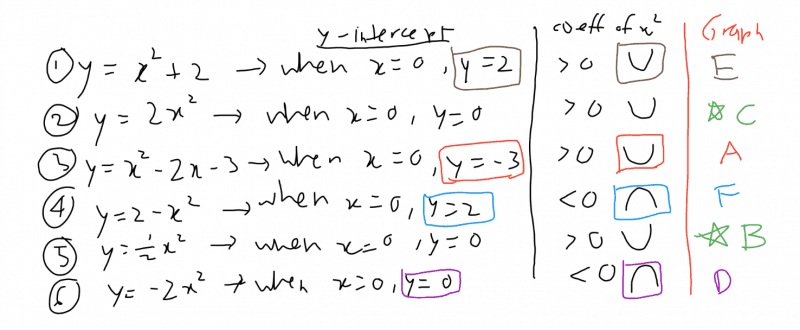# AnswerHi, based on y intercept and coefficient of x^2 to determine the shape of the curve, 4 of the graphs could be easily identified (A, D, E, F)

We are thus left with graph B and C where both has same y-intercept and shape of the curve. To decide, we look at the coefficient of x^2. The bigger the coefficient of x^2, the steeper the graph will be. E.g
1) y = 2 (x^2) -> when x = 1, y = 2. when x = 2, y =4

2) y= 0.5 (x^2) -> when x = 1, y = 0.5. when x=2, y =2

Thus, y = 2(x^2) will belongs to graph C and

y = 0.5 (x^2) will belongs to graph B.

Hope it helps! Pls help to click on “Accept Answer” link and like the answer if the answer is of help to you. Thanks! 🙂

0 Replies 0 Likes ✔Accepted Answer